# Maharashtra SSC Board (MSBSHSE) Question Paper for Class 10th Science Paper 1 2017 In PDF

## MSBSHSE 10th Standard Science Paper 1 Exam Question Papers 2017 with Solutions – Free Download

Students can very easily earn full scores in the MSBSHSE Class 10 Science I exam if they know the complex formulas and equations from Science. Students are advised to revise the subject by answering the previous papers of msbshse class 10 science. This will help them to gain thorough knowledge of the exam pattern. This will also help the students to get familiar with different types of questions and gauge the difficulty level of questions that are likely to be asked in the board exams.

### MSBSHSE Class 10 Science I 2017 Question Paper with Solutions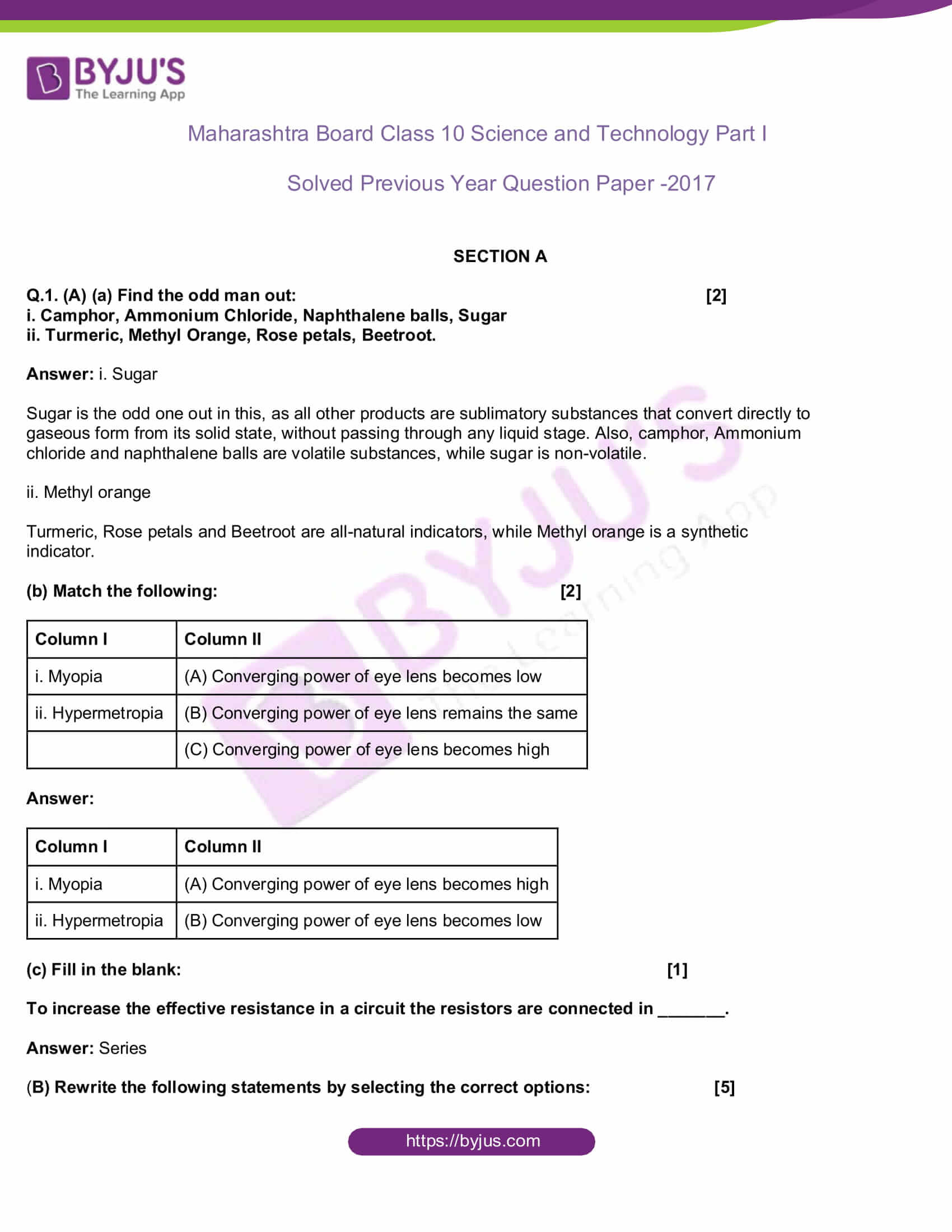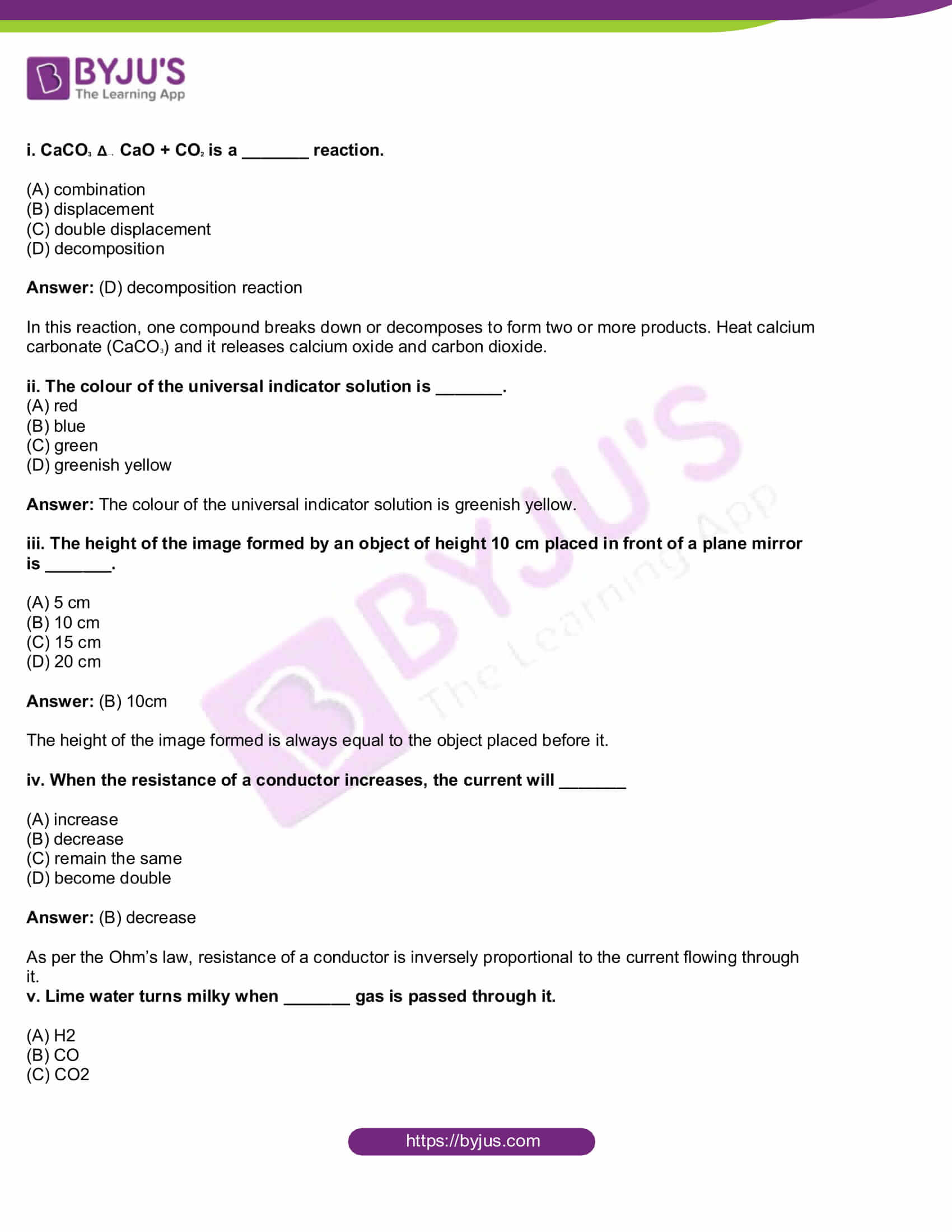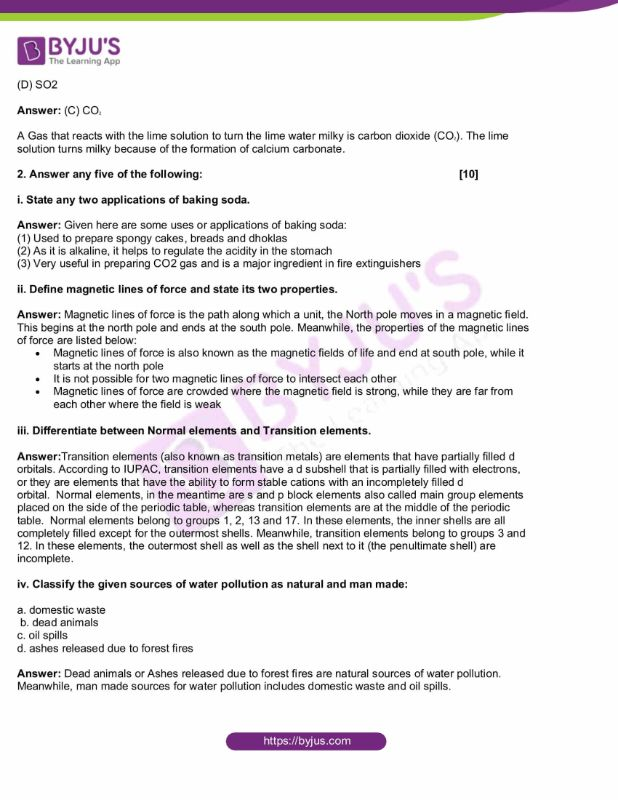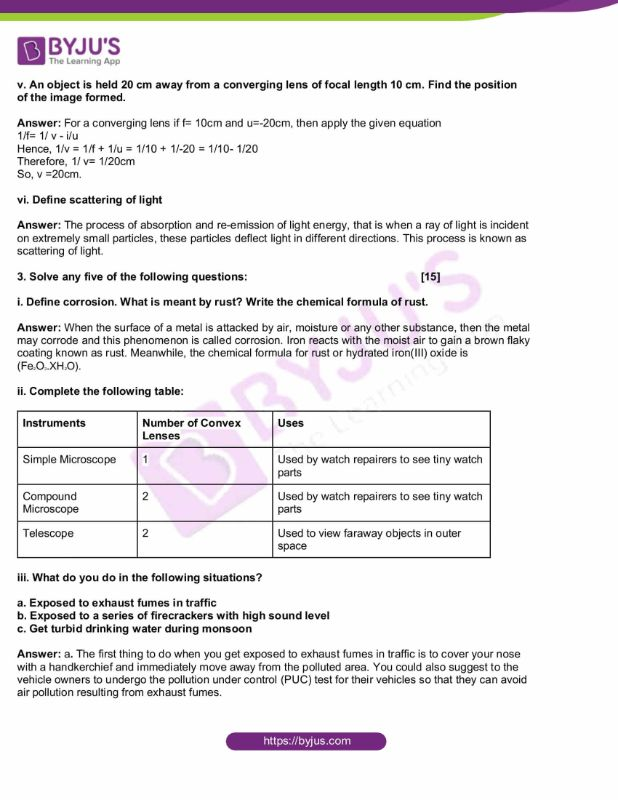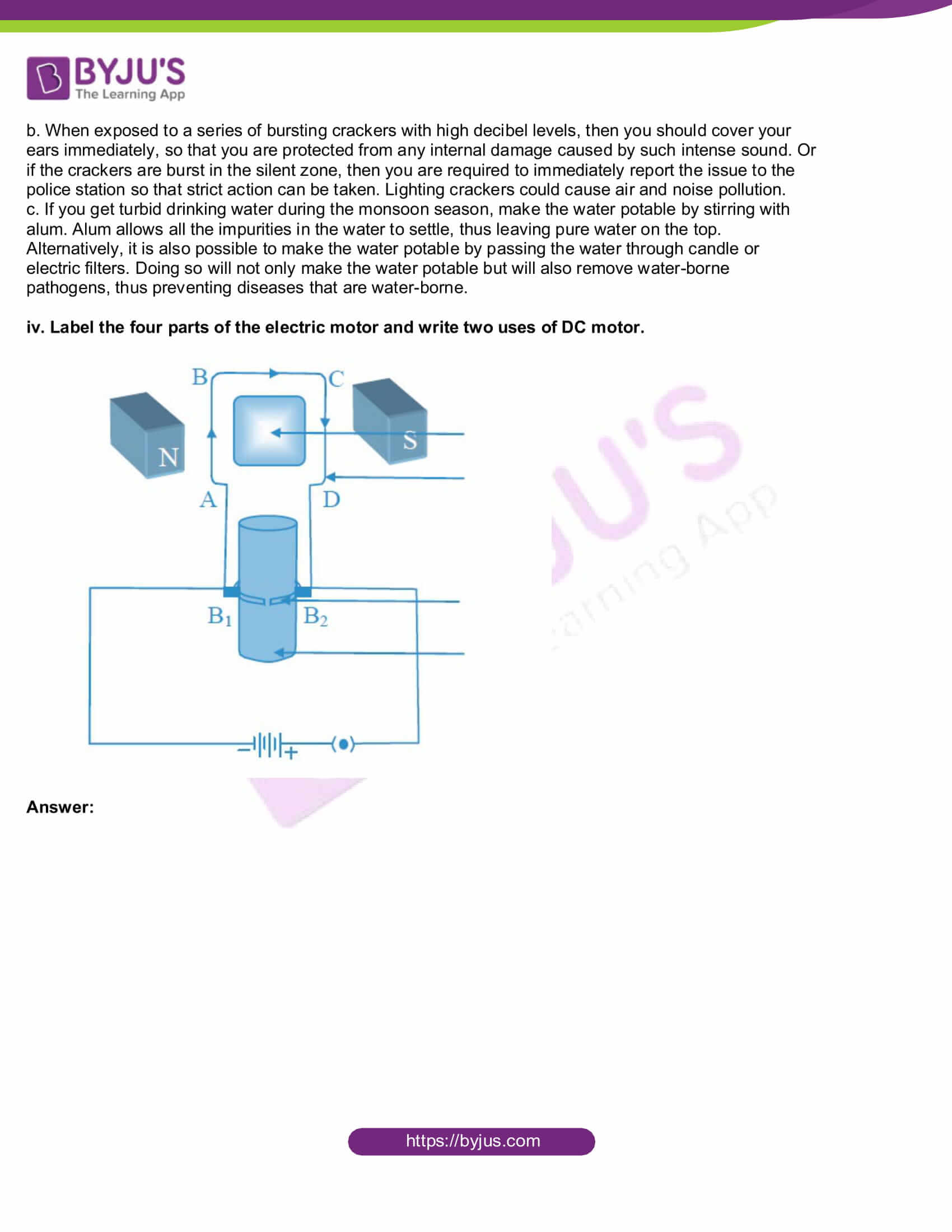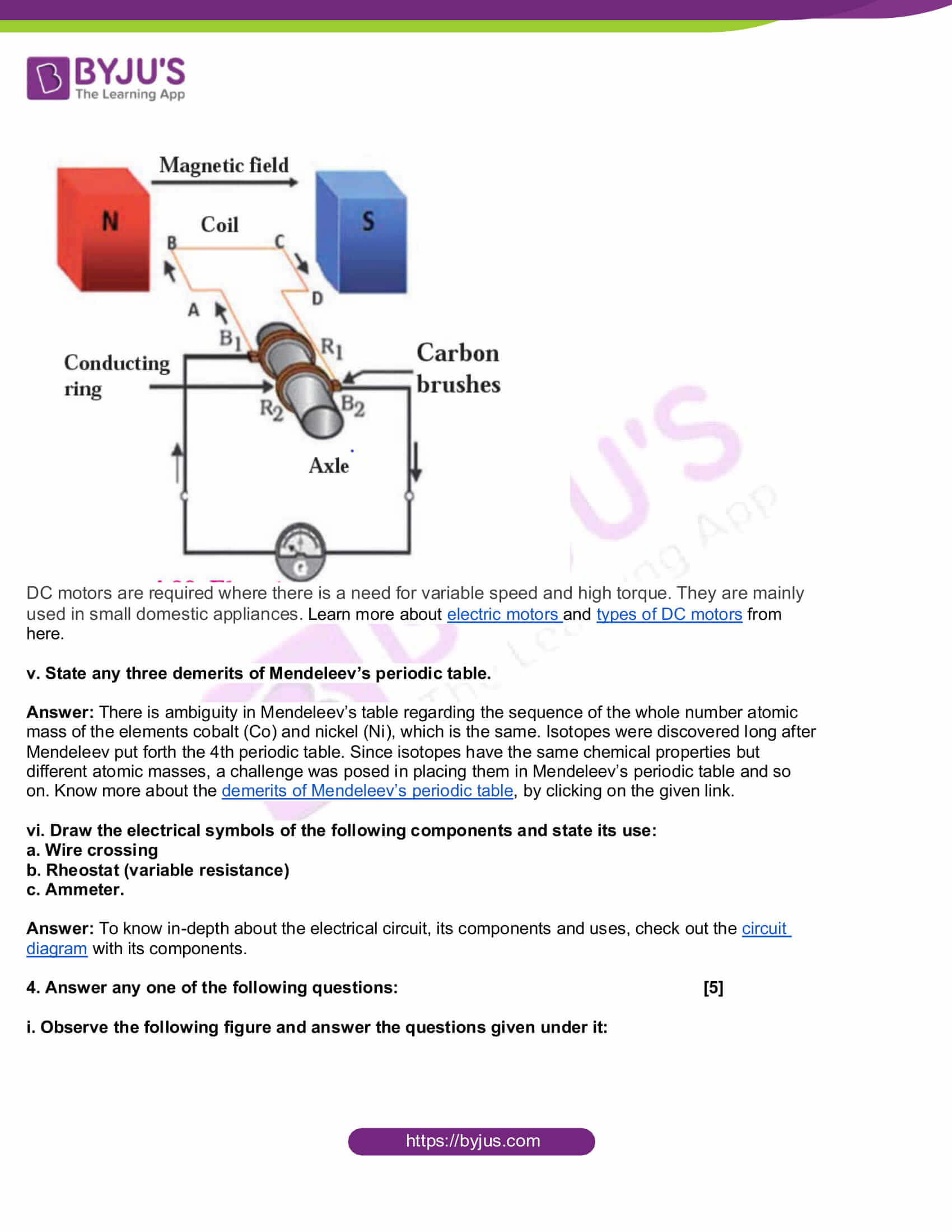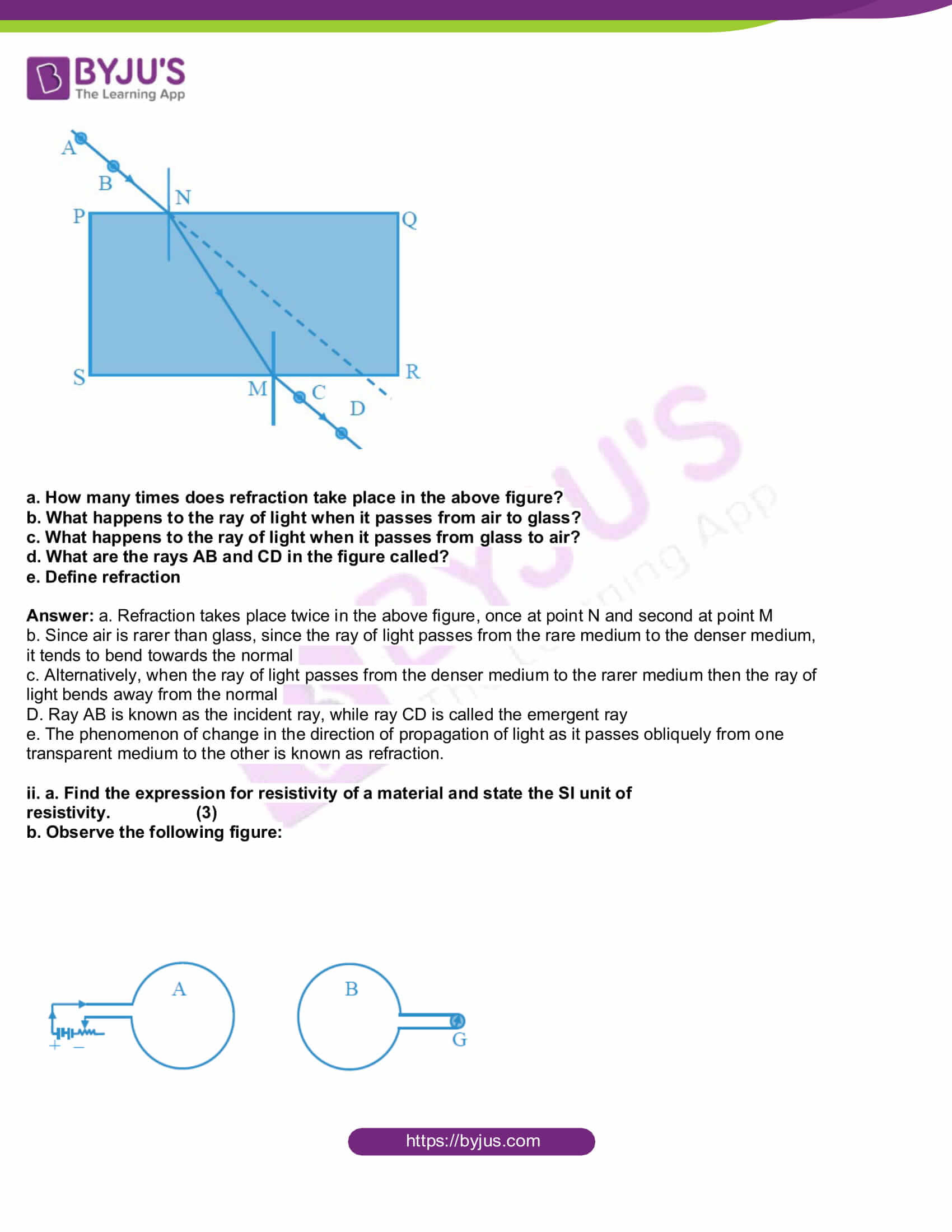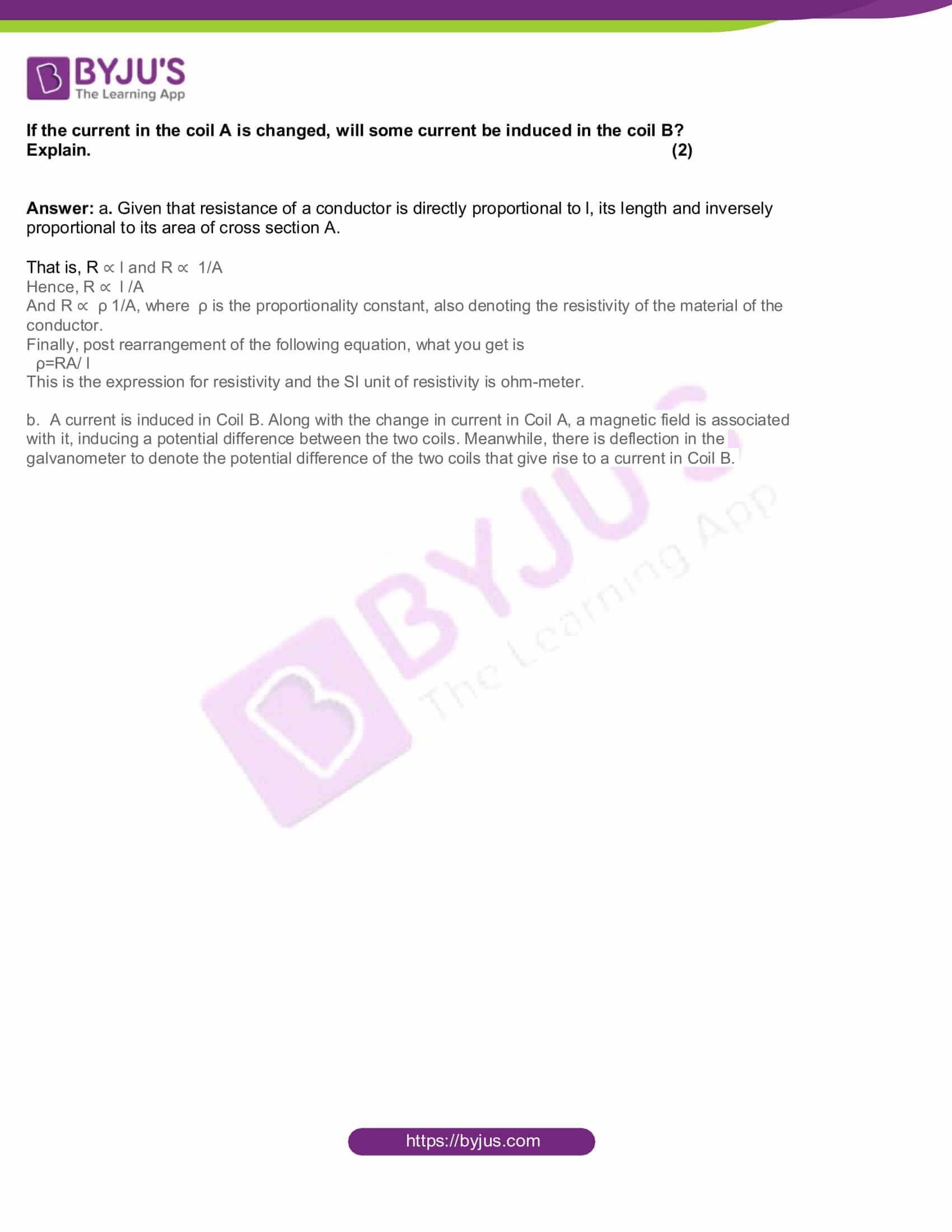SECTION A

Q.1. (A) (a) Find the odd man out: 

i. Camphor, Ammonium Chloride, Naphthalene balls, Sugar

ii. Turmeric, Methyl Orange, Rose petals, Beetroot.

Sugar is the odd one out in this, as all other products are sublimatory substances that convert directly to gaseous form from its solid state, without passing through any liquid stage. Also, camphor, Ammonium chloride and naphthalene balls are volatile substances, while sugar is non-volatile.

ii. Methyl orange

Turmeric, Rose petals and Beetroot are all natural indicators, while Methyl orange is a synthetic indicator.

(b) Match the following: 

 Column I Column II i. Myopia (A) Converging power of eye lens becomes low ii. Hypermetropia (B) Converging power of eye lens remains the same (C) Converging power of eye lens becomes high

 Column I Column II i. Myopia (A) Converging power of eye lens becomes high ii. Hypermetropia (B) Converging power of eye lens becomes low

(c) Fill in the blank: 

To increase the effective resistance in a circuit the resistors are connected in _______.

(B) Rewrite the following statements by selecting the correct options: 

i. CaCO3 Δ CaO + CO2 is a _______ reaction.

(A) combination

(B) displacement

(C) double displacement

(D) decomposition

In this reaction, one compound breaks down or decomposes to form two or more products. Heat calcium carbonate (CaCO3) and it releases calcium oxide and carbon dioxide.

ii. The colour of the universal indicator solution is _______.

(A) red

(B) blue

(C) green

(D) greenish yellow

Answer: The colour of the universal indicator solution is greenish yellow.

iii. The height of the image formed by an object of height 10 cm placed in front of a plane mirror is _______.

(A) 5 cm

(B) 10 cm

(C) 15 cm

(D) 20 cm

The height of the image formed is always equal to the object placed before it.

iv. When the resistance of a conductor increases, the current will _______

(A) increase

(B) decrease

(C) remain the same

(D) become double

As per the Ohm’s law, resistance of a conductor is inversely proportional to the current flowing through it.

v. Lime water turns milky when _______ gas is passed through it.

(A) H2

(B) CO

(C) CO2

(D) SO2

A Gas that reacts with the lime solution to turn the lime water milky is carbon dioxide (CO2). The lime solution turns milky because of the formation of calcium carbonate.

2. Answer any five of the following: 

i. State any two applications of baking soda.

Answer: Given here are some uses or applications of baking soda:

(1) Used to prepare spongy cakes, breads and dhoklas

(2) As it is alkaline, it helps to regulate the acidity in the stomach

(3) Very useful in preparing CO2 gas and is a major ingredient in fire extinguishers

ii. Define magnetic lines of force and state its two properties.

Answer: Magnetic lines of force is the path along which a unit, the North pole moves in a magnetic field. This begins at the north pole and ends at the south pole. Meanwhile, the properties of the magnetic lines of force are listed below:

• Magnetic lines of force is also known as the magnetic fields of life and end at south pole, while it starts at the north pole
• It is not possible for two magnetic lines of force to intersect each other
• Magnetic lines of force are crowded where the magnetic field is strong, while they are far from each other where the field is weak

iii. Differentiate between Normal elements and Transition elements.

Answer:Transition elements (also known as transition metals) are elements that have partially filled d orbitals. According to IUPAC, transition elements have a d subshell that is partially filled with electrons, or they are elements that have the ability to form stable cations with an incompletely filled d orbital. Normal elements, in the meantime are s and p block elements also called main group elements placed on the side of the periodic table, whereas transition elements are at the middle of the periodic table. Normal elements belong to groups 1, 2, 13 and 17. In these elements, the inner shells are all completely filled except for the outermost shells. Meanwhile, transition elements belong to groups 3 and 12. In these elements, the outermost shell as well as the shell next to it (the penultimate shell) are incomplete.

iv. Classify the given sources of water pollution as natural and man made:

a. domestic waste

c. oil spills

d. ashes released due to forest fires

Answer: Dead animals or Ashes released due to forest fires are natural sources of water pollution. Meanwhile, man made sources for water pollution includes domestic waste and oil spills.

v. An object is held 20 cm away from a converging lens of focal length 10 cm. Find the position of the image formed.

Answer: For a converging lens if f= 10cm and u=-20cm, then apply the given equation

1/f= 1/ v – i/u

Hence, 1/v = 1/f + 1/u = 1/10 + 1/-20 = 1/10- 1/20

Therefore, 1/ v= 1/20cm

So, v =20cm.

vi. Define scattering of light

Answer: The process of absorption and re-emission of light energy, that is when a ray of light is incident on extremely small particles, these particles deflect light in different directions. This process is known as scattering of light.

3. Solve any five of the following questions: 

i. Define corrosion. What is meant by rust? Write the chemical formula of rust.

Answer: When the surface of a metal is attacked by air, moisture or any other substance, then the metal may corrode and this phenomenon is called corrosion. Iron reacts with the moist air to gain a brown flaky coating known as rust. Meanwhile, the chemical formula for rust or hydrated iron(III) oxide is (Fe2O3.XH2O).

ii. Complete the following table:

 Instruments Number of Convex Lenses Uses Simple Microscope 1 Used by watch repairers to see tiny watch parts Compound Microscope 2 Used by watch repairers to see tiny watch parts Telescope 2 Used to view faraway objects in outer space

iii. What do you do in the following situations?

a. Exposed to exhaust fumes in traffic

b. Exposed to a series of firecrackers with high sound level

c. Get turbid drinking water during monsoon

Answer: a. The first thing to do when you get exposed to exhaust fumes in traffic is to cover your nose with a handkerchief and immediately move away from the polluted area. You could also suggest to the vehicle owners to undergo the pollution under control (PUC) test for their vehicles so that they can avoid air pollution resulting from exhaust fumes.

b. When exposed to a series of bursting crackers with high decibel levels, then you should cover your ears immediately, so that you are protected from any internal damage caused by such intense sound. Or if the crackers are burst in the silent zone, then you are required to immediately report the issue to the police station so that strict action can be taken. Lighting crackers could cause air and noise pollution.

c. If you get turbid drinking water during the monsoon season, make the water potable by stirring with alum. Alum allows all the impurities in the water to settle, thus leaving pure water on the top. Alternatively, it is also possible to make the water potable by passing the water through candle or electric filters. Doing so will not only make the water potable but will also remove water-borne pathogens, thus preventing diseases that are water-borne.

iv. Label the four parts of the electric motor and write two uses of DC motor.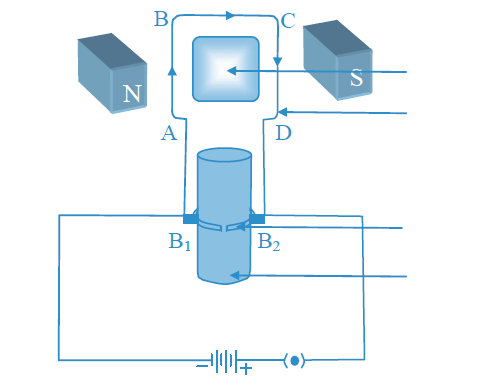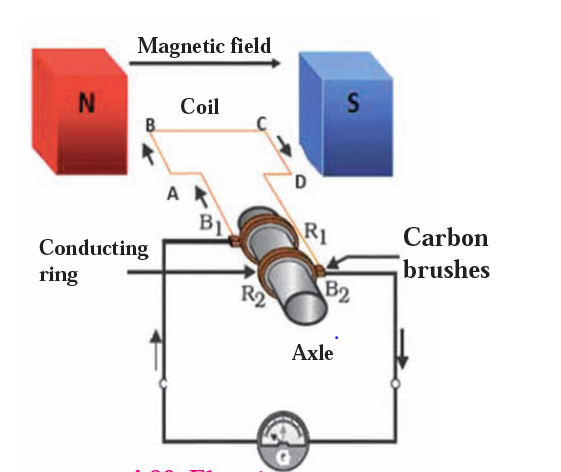DC motors are required where there is a need for variable speed and high torque. They are mainly used in small domestic appliances. Learn more about electric motors and types of DC motors from here.

v. State any three demerits of Mendeleev’s periodic table.

Answer: There is ambiguity in Mendeleev’s table regarding the sequence of the whole number atomic mass of the elements cobalt (Co) and nickel (Ni), which is the same. Isotopes were discovered long after Mendeleev put forth the 4th periodic table. Since isotopes have the same chemical properties but different atomic masses, a challenge was posed in placing them in Mendeleev’s periodic table and so on. Know more about the demerits of Mendeleev’s periodic table, by clicking on the given link.

vi. Draw the electrical symbols of the following components and state its use:

a. Wire crossing

b. Rheostat (variable resistance)

c. Ammeter.

Answer: To know in-depth about the electrical circuit, its components and uses, check out the circuit diagram with its components.

4. Answer any one of the following questions: 

i. Observe the following figure and answer the questions given under it: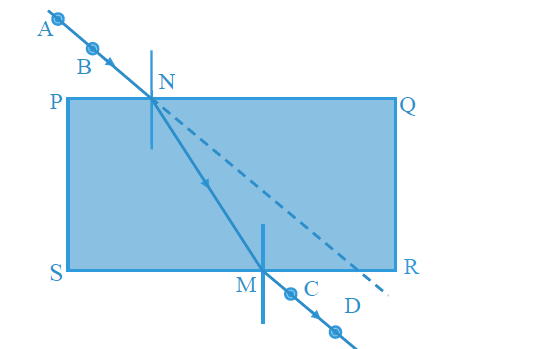a. How many times does refraction take place in the above figure?

b. What happens to the ray of light when it passes from air to glass?

c. What happens to the ray of light when it passes from glass to air?

d. What are the rays AB and CD in the figure called?

e. Define refraction

Answer: a. Refraction takes place twice in the above figure, once at point N and second at point M

b. Since air is rarer than glass, since the ray of light passes from the rare medium to the denser medium, it tends to bend towards the normal

c. Alternatively, when the ray of light passes from the denser medium to the rarer medium then the ray of light bends away from the normal

D. Ray AB is known as the incident ray, while ray CD is called the emergent ray

e. The phenomenon of change in the direction of propagation of light as it passes obliquely from one transparent medium to the other is known as refraction.

ii. a. Find the expression for resistivity of a material and state the SI unit of resistivity. (3)

b. Observe the following figure: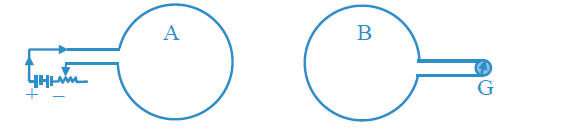If the current in the coil A is changed, will some current be induced in the coil B?

Explain. (2)

Answer: a. Given that resistance of a conductor is directly proportional to l, its length and inversely proportional to its area of cross section A.

That is, R ∝ l and R ∝ 1/A

Hence, R ∝ l /A

And R ∝ ρ 1/A, where ρ is the proportionality constant, also denoting the resistivity of the material of the conductor.

Finally, post rearrangement of the following equation, what you get is

ρ=RA/ l

This is the expression for resistivity and the SI unit of resistivity is ohm-meter.

b. A current is induced in Coil B. Along with the change in current in Coil A, a magnetic field is associated with it, inducing a potential difference between the two coils. Meanwhile, there is deflection in the galvanometer to denote the potential difference of the two coils that give rise to a current in Coil B.